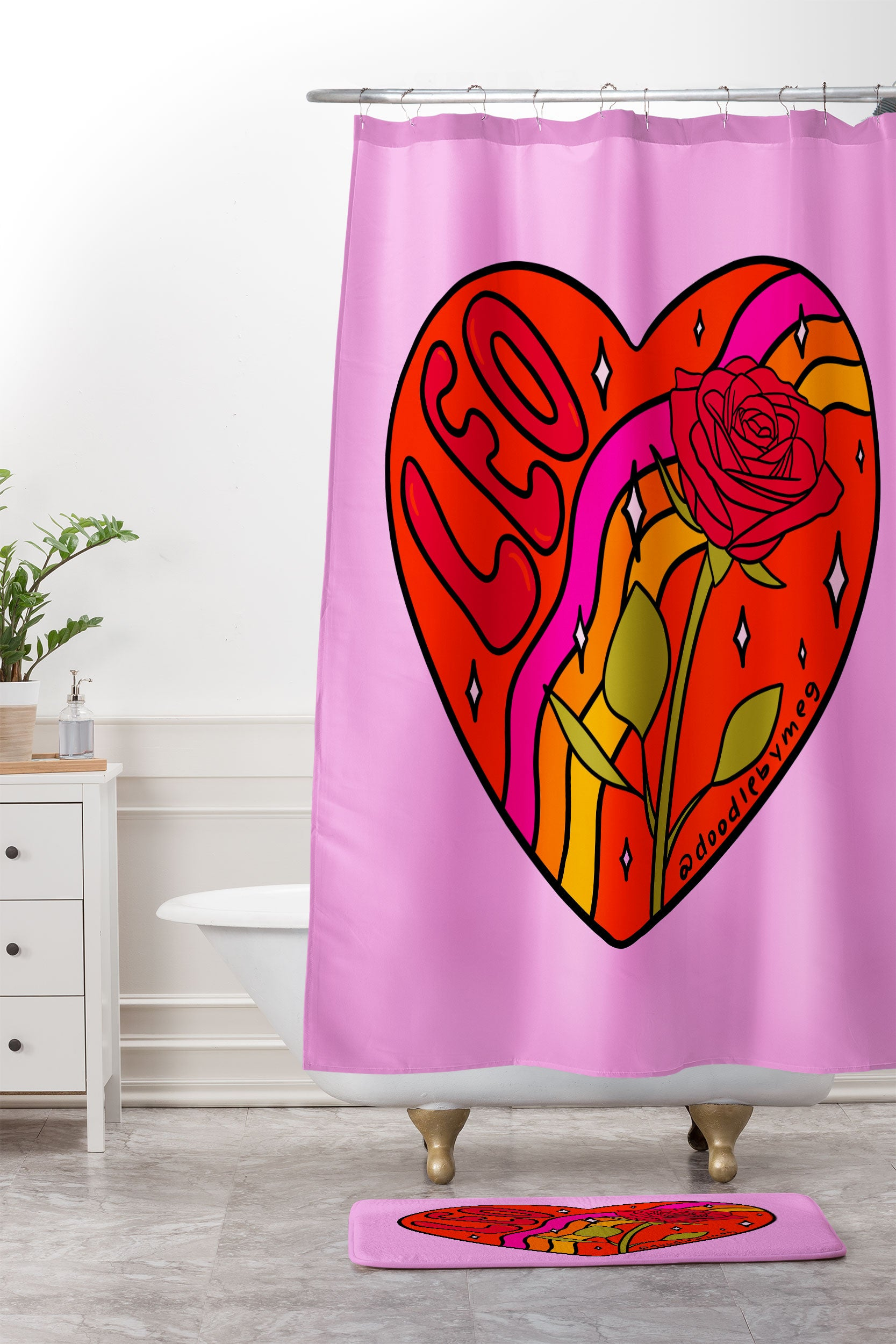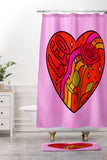# function singularize(input) { if(input == 'art canvas'){ return input } let inputArr = input.split('') if(inputArr[inputArr.length - 1] == 's' && inputArr[inputArr.length - 2] != 'e'){ inputArr.splice(inputArr.length-1, 1) return inputArr.join('') } else if(inputArr[inputArr.length - 1] == 's' && inputArr[inputArr.length - 2] == 'e' && inputArr[inputArr.length - 3] != 'i'){ inputArr.splice(inputArr.length-2, 2) return inputArr.join('') } else if(inputArr[inputArr.length - 1] == 's' && inputArr[inputArr.length - 2] == 'e' && inputArr[inputArr.length - 3] == 'i'){ inputArr.splice(inputArr.length-3, 3) inputArr.push('y') return inputArr.join('') } else {return input} } \$('.title').text(singularize('shower curtain and mat'))

leo valentine  BY doodle by meg
76198-showb1

DENY Doodle By Meg

Doodle By Meg Leo Valentine Shower Curtain And Mat

76198-showb1

\$154.00

MSRP: \$0.00Deny Designs is for wholesale customers only.To Top